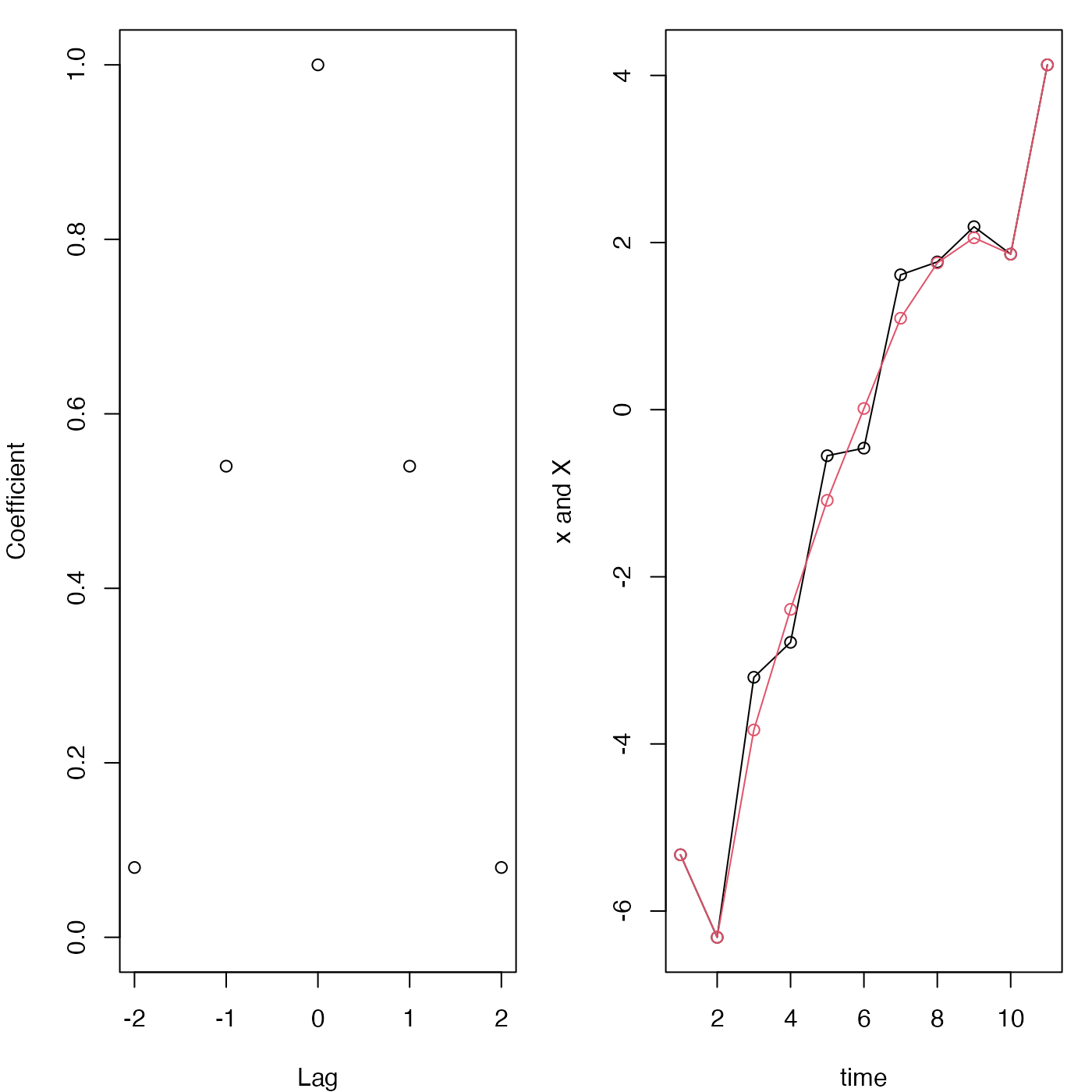The filter coefficients are constructed using standard definitions, and then filter in the stats package is used to filter the data. This leaves NA values within half the filter length of the ends of the time series, but these may be replaced with the original x values, if the argument replace is set to TRUE.

lowpass(x, filter = "hamming", n, replace = TRUE, coefficients = FALSE)

## Arguments

x a vector to be smoothed name of filter; at present, "hamming", "hanning", and "boxcar" are permitted. length of filter (must be an odd integer exceeding 1) a logical value indicating whether points near the ends of x should be copied into the end regions, replacing the NA values that would otherwise be placed there by stats::filter(). logical value indicating whether to return the filter coefficients, instead of the filtered values. In accordance with conventions in the literature, the returned values are not normalized to sum to 1, although of course that normalization is done in the actual filtering.

## Value

By default, lowpass returns a filtered version of x, but if coefficients is TRUE then it returns the filter coefficients.

## Caution

This function was added in June of 2017, and it may be extended during the rest of 2017. New arguments may appear between n and replace, so users are advised to call this function with named arguments, not positional arguments.

## Examples

library(oce)
par(mfrow=c(1, 2), mar=c(4, 4, 1, 1))
coef <- lowpass(n=5, coefficients=TRUE)
plot(-2:2, coef, ylim=c(0, 1), xlab="Lag", ylab="Coefficient")
x <- seq(-5, 5) + rnorm(11)
plot(1:11, x, type='o', xlab="time", ylab="x and X")X <- lowpass(x, n=5)
lines(1:11, X, col=2)points(1:11, X, col=2)